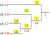# An Introduction to Coding Theory

Free!

language English Optional, paid

Error control coding is an indispensible part of any digital communication system. In this introductory course, we will discuss theory of linear block codes and convolutional codes, their encoding and decoding techniques as well as their applications in real world scenarios. Starting from simple repetition codes, we will discuss among other codes: Hamming codes, Reed Muller codes, low density parity check codes, and turbo codes. We will also study how from simple codes by concatenation we can build more powerful error correcting codes.

COURSE LAYOUT

Week 1-
Lecture 1: Introduction to error control coding
Lecture 2: Introduction to linear block codes, generator matrix and parity check matrix
Lecture 3: Properties of linear block codes: Syndrome, error detection
Week 2-
Lecture 4: Decoding of linear block codes
Lecture 5: Distance properties of linear block codes
Week 3-
Lecture 6: Some simple linear block codes: Repetition codes, Single parity check codes, Hamming codes, Reed Muller codes
Lecture 7: Bounds on size of codes: Hamming bound, Singleton bound, Plotkin bound, Gilbert-Varshamov bound
Week 4-
Lecture 8: Introduction to convolutional codes-I: Encoding, state diagram, trellis diagram
Lecture 9: Introduction to convolutional codes-II: Classification, realization, distance properties
Lecture 10: Decoding of convolutional codes-I: Viterbi algorithm
Week 5-
Lecture 11: Decoding of convolutional codes-II: BCJR algorithm
Lecture 12: Performance bounds for convolutional codes
Week 6-
Lecture 13: Low density parity check codes
Lecture 14: Decoding of low density parity check codes: Belief propagation algorithm on BSC and AWGN channels
Week 7-
Lecture 15: Turbo codes
Lecture 16: Turbo decoding
Week 8-
Lecture 17: Distance properties of turbo codes
Lecture 18: Convergence of turbo codes
Lecture 19: Automatic repeat request schemes
Lecture 20: Applications of linear codes.

## User Reviews

0.0 out of 5
0
0
0
0
0

There are no reviews yet.

×